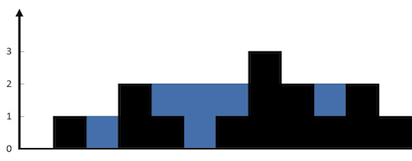# GeetCode Hub

Given `n` non-negative integers representing an elevation map where the width of each bar is `1`, compute how much water it can trap after raining.

Example 1:```Input: height = [0,1,0,2,1,0,1,3,2,1,2,1]
Output: 6
Explanation: The above elevation map (black section) is represented by array [0,1,0,2,1,0,1,3,2,1,2,1]. In this case, 6 units of rain water (blue section) are being trapped.
```

Example 2:

```Input: height = [4,2,0,3,2,5]
Output: 9
```

Constraints:

• `n == height.length`
• `0 <= n <= 3 * 104`
• `0 <= height[i] <= 105`

class Solution { public int trap(int[] height) { } }